# Locating and Sizing of Distributed Generation Sources and Parallel Capacitors Using Multiple Objective Particle Swarm Optimization Algorithm

Locating and Sizing of Distributed Generation Sources and Parallel Capacitors Using Multiple Objective Particle Swarm Optimization Algorithm

Mehrdad Ahmadi KamarposhtiGiulio Lorenzini Ahmed Amin Ahmed Solyman

Department of Electrical Engineering, Jouybar Branch, Islamic Azad University, Jouybar, Iran

Department of Industrial Engineering, University of Parma, Parco Area Delle Science 181/A, Parma 43124, Italy

Department of Electrical and Electronic Engineering, Istanbul Gelisim University, Avcilar, Turkey

Corresponding Author Email:
mehrdad.ahmadi.k@gmail.com
Page:
10-24
|
DOI:
https://doi.org/10.18280/mmep.080102
Received:
29 September 2020
|
Accepted:
13 January 2021
|
Published:
28 February 2021
| Citation

OPEN ACCESS

Abstract:

In this paper, the MOPSO algorithm has been used to locate and determine the capacity of distributed generation sources and capacitor banks in the distribution system. The intended objective function is a combination of different objective functions. The first goal is to reduce losses, and the second goal is to improve the voltage profile and the third goal is to reduce costs, which has been used by placing weight coefficients in the form of an objective function in the algorithms. For this purpose, the standard 33-bus system has been used to conduct studies. Studies have been repeated in three scenarios. In the first scenario, the locating and determination of the capacity of active and reactive resources has been accomplished with the approach of reducing losses and improving the voltage profile. However, in the second scenario, the locating and determining the capacity of these resources has been accomplished with loss and cost reduction approach and it was considered as constraint in voltage profile. In the third scenario, the simultaneous reduction of all three objective functions has been performed simultaneously. To validate the results obtained by the MOPSO algorithm, its results were compared with genetic and particle swarm algorithms. The results indicate better and more accurate performance of MOPSO algorithm in minimizing objective functions relative to other two algorithms.

Keywords:

distributed generation, parallel capacitors, voltage profile, loss reduction, MOPSO algorithm

1. Introduction

In interconnected power systems, with economies of scale, electric power generation is centralized by large power plants. In the early years of the emergence of interconnected systems, the system typically has an annual energy consumption of about six to seven percent of electric power consumption. In the 1970s, debates such as the oil crisis and environmental issues raised new problems for the electricity industry, as in the 1980s, these factors and economic changes led to a drop in load growth to around 1.6 to 3 percent per year. At the same time, the cost of transmission and distribution of electrical energy has increased significantly. Therefore, central power generation by large power plants was often impracticable due to reduced load growth, increased transmission and distribution costs, environmental degradation, and technological change and various legislation.

The constraints on energy resources and cost-effective energy transfer have encouraged engineers, designers and researchers in the electricity distribution industry to increase efficiency and reduce network losses over the past decade. In other words, an increase in the reduction in loss will lead to an increase in capital. Hence, loss reduction is an economic activity and reduces the cost of installing additional equipment in the network, the rate of energy and power loss, carbon dioxide emission rate and other economic parameters. About 75% of the network loss occurs in the distribution sector, so loss in distribution systems are of great importance. Reconstruction, capacitor placement and the use of distributed generation sources are of the effective ways to reduce loss and improve the voltage profile in distribution networks, which draw nowadays much more attention.

The use of distributed generation (DG) to generate electricity is one of the ways that today is being proposed to provide consumer power. Generating electricity near the place of consumption, in addition to reducing the losses in the system, can provide more flexibility to provide a variety of services to consumers. In fact, the micro-network is a collection of loads and generators which can work in island form or connected to the network. Micro-network generators are generally in the form of distributed resource generators due to low load and the nature of the micro-network. These resources can be solar cells, wind turbines, micro turbines, batteries, and more.

The use of DG in power systems can provide benefits such as power quality, improved voltage profiles, loss reduction and peak shaving, increased reliability and reduced line congestion. All of these benefits depend on the size and installation location of the DG. Otherwise, not only will the above benefits not be met it also increases total losses and reduces the voltage at the bus position and increases the congestion of the transmission line. In addition to the benefits mentioned, the small size of these resources is another advantage which makes it possible to install and operate in a very short time at the desired location.

The use of small generators to generate electricity was lost after the creation of large power plants but with the advent of small-scale power generation technologies and the restructuring of the electric power industry and environmental issues, it has led to the re-emergence of these generators in the electricity industry. If distributed sources in distribution networks are installed at the right place with optimal capacity, this will improve the technical and economic parameters of this network.

Active and reactive resources can benefit distributed generations. But given that the production of reactive power by capacitors at a lower cost is possible, the power factor of the energy generated by distributed generation can be considered in optimal mode equal to unit one and distributed generation can be modeled as PQ busbar and net active power generator.

One of the ways today to generate power is to provide consumer power. Generating electricity near the place of consumption, in addition to reducing the losses in the system, can provide more flexibility to provide a variety of services to consumers. The use of DG in power systems can provide benefits such as: power quality, improved voltage profiles, loss reduction and peak shaving, increased reliability and reduced line congestion. All of these benefits depend on the size and installation location of the DG. Otherwise, not only will the above benefits not be met it also increases total losses and reduces the voltage at the bus position and increases the congestion of the transmission line. In addition to the benefits mentioned, the small size of these resources is another advantage which makes it possible to install and operate in a very short time at the desired location. The use of small generators to generate electricity was lost after the creation of large power plants but with the advent of small-scale power generation technologies and the restructuring of the electric power industry and environmental issues, it has led to the re-emergence of these generators in the electricity industry. If distributed sources in distribution networks are installed at the right place with optimal capacity, this will improve the technical and economic parameters of this network.

Active and reactive resources can benefit distributed generations. But given that the production of reactive power by capacitors at a lower cost is possible, the power factor of the energy generated by distributed generation can be considered in optimal mode equal to unit one and distributed generation can be modeled as PQ busbar and net active power generator. With active and reactive power supply of loads in the load location, in addition to reducing losses due to power transmission, the voltage profile can be maintained within the permissible range, therefore these two reliability indicators are improved. But the issue that matters most is the amount of active and reactive power required by the selected bus. Because the lack of proper selection of each of these values will have a high cost and low efficiency .

In this paper, the MOPSO multi-objective algorithm will be used to determine the optimal capacities of the capacitor and distributed generations in the standard network to improve the bus voltage profile, reduce line losses and reduce costs simultaneously. All of these benefits depend on the size and installation location of the DG. Otherwise, not only will the above benefits not be met it also increases total losses and reduces the voltage at the bus position and increases the congestion of the transmission line.

2. An Overview of the Research Background

The optimal locating of distributed generation sources and capacitor placement are both topics that have been studied on a large scale. Many articles have examined these two subjects separately. Few references have both considered simultaneously into the optimization problem. These references are different with each other in how to define the objective function (single-objective or multi-objective) and the type of optimization method used to solve the problem. In this section, first, references that address the optimal locating of distributed generation sources are examined, and then the references about capacitor placement have been addressed. Subsequently, studies that have considered the "optimal locating of distributed generation sources" and "capacitor placement" simultaneously.

2.1 An overview of the research background in the optimal locating of distributed generation sources

In most cases examined, probabilistic and experimental methods have been used to achieve the objective. The objective functions are different in the examined cases; but in all of them, it is possible to achieve the objectives by using the optimal locating of distributed generation sources in the network. Therefore, that power network metrics are met.

The location of DGs is determined and the load is variable with time, the amount of power generated by DGs is unknown . In this paper, a linear programming method has been used which are mathematical and numerical optimization methods. Due to the fact that the capacity of distributed products has not been specified, the use of this method is not time consuming; but if the amount of DG generation is also needed, this method will not be responsive.

A new hybrid method is proposed by Gomez et al.  that uses discrete particle swarm optimization and optimal power flow (OPF). Technical constraints are also used in the optimization process to search the best locations to link distributed generation sources to the distribution network. The results obtained from the proposed algorithm and the genetic algorithm have been compared which suggests that the proposed method provides a more optimal response.

A combination method of GA and PSO algorithms for locating distributed generation sources has been proposed by Moradi and Abedini  to achieve the minimum losses, increase voltage stability and improve the voltage regulation index.

A comparison between power dissipation sensitivity, power stability index (PSI), and voltage stability index (VSI) has been proposed by Murty and Kumar  to determine the optimal location and size of distributed generation units in the radial distribution network. The main sections of this article are:

• Optimal location determination of distributed generation sources based on new methods of power dissipation and PSI sensitivity.
• The voltage stability index method proposed for optimal locating of distributed generation units.
• Comparison of sensitivity methods in the optimal locating of distributed generation sources and determination of their size.
• Optimal location determination of distributed generation sources considering load growth.
• The effect of distributed generation sources in the combination of power factor coefficients.
• The effect of distributed generation sources on the improvement of the margin of voltage stability.

The quasi-opposition-training-learning-based-optimization (QOTLBO) method is used to find the optimal location of distributed generation sources and simultaneously power loss minimization, improved voltage stability index, and the radial distribution network voltage deviation will be investigated . The simulation results of the proposed method are compared with other methods of artificial intelligence such as GA/PSO, GA, PSO and thermal simulation loss sensitivity coefficient are compared. The results show that the QOTLBO method is superior to other methods.

Various distributed generation sources for active and reactive power compensation have been investigated in order to minimize power losses in distribution networks using the PSO algorithm . Also, the other goals in this paper are to improve the voltage profile of all the buses. Studies have been done on standard 33 and 69-bus IEEE systems and the results obtained by the proposed method in this reference have been compared with analytical methods. In this reference, it has been claimed that in larger systems, metaheuristic methods are more suitable due to faster convergence.

A repetitive load distribution method has been used to calculate power losses in the system . The type of distributed generation used in this paper is photovoltaic. The purpose of the determination of location and photovoltaic capacity was to reduce power losses and improve the voltage profile. In addition to the locating of distributed generation sources, the system has also been rearranged.

An analytical method has been used to determine the size and location of photovoltaic panels based on massive placement on the primary side of the distribution system . The main goal of this research is to reduce power losses and improve the voltage profile with economic benefits. Finally, it has been shown that the massive placement of distributed generation production units in comparison with single mode leads to improved research goals.

A multi-purpose optimization method is used to determine optimal location of distributed wind and solar generation units in the distribution system based on the PSO approach . The proposed method follows the voltage and power range set by the power company. The purpose of this method is to improve the voltage stability and reduce power losses of power grids by distributed generation units. A new voltage stability factor (VSF) is used to measure the voltage stability level in different network busbars. VSF is also used to assess the voltage stability status throughout the network. Compared to other voltage stability indicators, it can be concluded that VSF development is very effective and simple. The choice of optimal size and location of wind units and photovoltaic arrays in distributed systems has been discussed and the optimal placement of this inverter between photovoltaic arrays and wind units is considered. It can be seen that the proposed method is more efficient than other techniques for placing DG in both fields to minimize losses and improve voltage stability. In addition, both wind and photovoltaic units are considered as active and reactive power sources in different power ratios. By analyzing the results it can be concluded that, the distributed wind and solar sources in the post-phase power factor increase the voltage profile in the distribution busbars. The results also show that the busbar voltage increases with increasing penetration of distributed generation units.

The authors presented an appropriate analytical method for determining four types of distributed generation units to reduce the losses in the primary distribution networks . This improved analytical method is a tool to calculate the optimal size of four different types of DGs and a methodology to determine the appropriate location for them. A method was also proposed for achieving the optimal power factor for delivering active and reactive power by DGs. In addition, the loss sensitivity factor (LSF) and Exhaustive Load Flow (ELF) are the methods presented. The analytical method has been tested experimentally on three different distribution systems with varying sizes and complexities. The results show that the analytical method is more effective than ELF and LSF responses. Despite its simplicity, analytical methods are not able to cope with optimization issues regarding the optimal allocation of DGs in unbalanced distribution networks due to the complexity of problems.

An efficient rapid convergence optimization algorithm has been presented based on the correction of the traditional firefighting method to determine the optimal size and location of distributed generation sources of controlled voltage in balanced and unbalanced distribution systems . The proposed algorithm has modified the traditional firefighting method to effectively limit the optimization problems by providing formulas for adjusting the parameters of the algorithm and updating the equations. The proposed algorithm is a tool in the MATLAB environment and on the IEEE standard networks to minimize system power losses by precisely identifying the optimal location and size of distributed generation units without undermining the system constraints. This method is used in balanced and unbalanced distribution feeders and the accuracy of the proposed method is checked by comparing the results obtained by it and other methods. The results show that the proposed method is more effective in determining DG size, this can be stated that the proposed algorithm can not only determine the optimal DG power efficiency but also can determine the optimal DG power reactive in the appropriate range which can hold the bus voltage at a specified voltage of 1 p.u.

A new metaheuristic method called Stud Krill Herd has been used for locating and determination of the capacity of distributed resources . Its main purpose is to reduce line losses by considering various constraints such as voltage range, actual generating power range of DG, power balance range and DG location range. The proposed method is implemented on the 33 and 69-bus system and can be used for any number of DGs, but for more certainty, the DG number has been considered to be 3. This method determines the optimal capacity and location of DGs in three basic load states, nominal load, and peak load finally, the results show that the proposed method is more favorable than other available methods.

The optimal location of a distributed 2.3 MW generation source in a standard 14-bus network is presented based on power losses . The optimal production location of the distributed generation will be determined by the power losses in each bus to which the DG is connected. Power losses per bus are obtained by Neplan software using the Newton-Raphson method. Distributed generation can play an important role in improving reliability, power quality and voltage stability in addition to reducing losses. Finally, the results show that the optimal location to reduce the losses in the system under study is in the bus 14.

The FPA algorithm is used to determine the optimal location and capacity of the distributed generation units in order to reduce the actual system power losses and improve the bus voltages in radial distribution networks with 33, 69 and 136 buses . For validation, the proposed algorithm has been compared with other metaheuristic optimization algorithms such as ABC and CSA and the results show that research goals have improved with this algorithm.

2.2 An overview of the research background in the optimal capacitor locating

A large percentage of power system losses occur in the distribution network. Capacitor placement is one of the common methods for reducing reactive current in lines and, consequently, reducing losses. Power factor correction and voltage profile improvement are also other advantages of capacitor placement.

Optimum capacitor placement has been investigated from the 1960s. Many algorithms have been proposed to solve this problem, the early algorithms have been more heuristic and they have considered the issue as simpler. In other words, some of the problem arguments were not considered.

In the 1980s, the problem was examined more thoroughly and more comprehensively. For example, a voltage-dependent model has been proposed that considers voltage variations along the feeder . The location of the capacitors also changes as a continuous variable from the beginning of the feeder.

The authors have modeled the problem as a non-linear programming problem mixed with integer numbers . There, the problem has been approximated by a derivative function that can be solved by the Benders decomposition method.

In the 1990s, solving method through hybrid algorithms have been presented. Among the proposed methods, metaheuristic algorithms have a special place, because accurate optimization algorithms are usually not suitable for solving real problems. The taboo search method is used to solve the problem . There, load growth, feeder capacity and voltage limits have been considered to minimize investment costs and system energy losses. Also, a sensitivity analysis method has been used to identify selected locations for capacitor placement and this will reduce the search space of the problem. Compared to the SA method, the superiority of the TS method has been shown.

The problem of locating and measuring a capacitor using a genetic algorithm has been solved . Here is also a sensitivity analysis method that identifies selected capacitor placement locations and reduces the search space.

A new method for locating the capacitor has been proposed which uses a particle swarm algorithm with operators based on the Gaussian and Cauchy probability functions . The proposed method is proved by two examples. Simulation results show that the proposed method can achieve a very optimal solution on the overall search with very little calculated time.

In the method proposed by Devabalaji et al.  an integrated approach of the loss sensitivity factor (LSF) and voltage stability index (VSI) is used to determine the optimal location of the connection of capacitive banks. In this paper, an optimization algorithm for bacterial nutrition has been used to determine the optimal size of the capacitors. The proposed method applies to a 34-busbar radial distribution network of 85 IEEE standard busbars. The simulation results show the performance and effectiveness of the proposed method.

Taher and Bagherpour  proposed a honey bee hybrid optimization algorithm for locating the shunt capacitors in the 25-bus and 27-bus IEEE standard distribution system. The objective function includes minimizing the loss and maintaining the total harmonic distortion (THD).

Two new algorithms are used to solve the problem of optimal locating of capacitors in a radial distribution system using two methods: Optimal locating of fixed capacitor banks (variable locations of fixed capacitive banks-VLFQ) and optimal size and capacitor locating (Variable Locations Variable sizing of Capacitors-VLVQ) are used to minimize power losses and maximize network resources . Two algorithms inspired by the nature of the bat algorithm (BA) and Coco search (CS): Search for all possible locations in the system with capacitors of different sizes, in which optimal capacitor sizes are selected based on standard sizes available on the market. In order to validate, the proposed algorithms apply to the standard 34 and 85-busbar radial distribution network and the results obtained from methods such as particle swarm optimization, harmonic search, genetic algorithm, artificial bee colony, training-learning optimization and plant growth simulation algorithm that will be available in other articles will be compared. The proposed method offers a high quality response and greater convergence speed.

Two methods are: Sensitivity Analysis and Gravity Search Algorithms (GSA) . Sensitivity analysis is a systematic technique used to reduce the search space and to find the right solution for determining the location of capacitors. Capacitor values are determined for the corresponding locations using the GSA algorithm. A number of networks with different situations have been used in different sizes and complexities in order to validate the proposed method. It is also compared with the internal point analytic algorithm and one of the metaheuristic methods called thermal simulation. The results show that the proposed method is able to provide more optimal responses.

Training-learning-based algorithm (TLBO) minimizes energy losses and costs by optimally locating the capacitors in the radial distribution system . The proposed algorithm examines the two learning aspects that call training phase and learning phase. For validation, the proposed method applies to radial distribution systems with 22, 69, 85, and 141 busbars. By comparing the proposed method with algorithms such as genetics, particle swarm, direct search algorithms, and integer linear programming methods, it is observed that, the results obtained from the proposed method provide better quality solutions.

2.3 An overview of the research background in the optimal locating of distributed generation sources and capacitors simultaneously

So far, a number of references have been investigated with the subject of optimal locating of distributed generation sources or capacitor. A number of references have also taken these two simultaneously. From the point of view of network utilization, the issue of the location of distributed generation sources and capacitors is proposed to minimize losses. From the perspective of mid-term and long-term planning, the locating of distributed generation sources and capacitor can be considered as the minimization of total costs including investment costs, cost of network losses, and so forth.

The locating of capacitor and distributed generation sources in the radial distribution network have been carried out simultaneously at different load levels . The goals are to reduce active and reactive power losses, reduce energy losses and improve the voltage profile. Also, the effect of capacitors and distributed generation sources on the improvement of voltage stability will be examined. The memetic algorithm is used to find optimal solutions. This algorithm is a combination of local search and genetic algorithm. The function of the proposed method runs on a sample distribution network.

A method has been proposed based on an analytical approach for determining the location and size of distributed generation sources and capacitors in order to reduce losses, taking into account the equal and unequal constraints of the distribution network . The sensitivity analysis method is used to identify candidate locations for distributed generation sources and capacitor locating. For validation, the proposed method has been applied to the standard distribution network with 12 and 33 busbars. The results of the optimization and comparison of the published studies indicate the location and size of the distributed generation sources and capacitors. By applying this method, loss reduction will be achieved with optimal voltage profile and also decrease in line load in distribution networks.

Gallano and Nerves  includes optimization studies with three objectives in the distribution system, namely: Minimizing power losses, improving voltage profiles and reducing line load by network redesign, capacitor locating and distributed generation sources are simultaneously performed. A new and fast multi-objective method that can be applied to a real network has been used. The proportionate non-dominated sorting genetic algorithm (NSGA-II) and fuzzy decision making analysis are used to obtain the best structure of the network by simultaneously connecting capacitors and distributed generation sources. The results show that in case of connecting capacitors and distributed generation sources, a more favorable response is obtained in comparison with the state in which these equipment is not present.

Optimal locating of capacitors and distributed generation sources is used to control the active and reactive power distribution . This paper is based on three improved optimization algorithms, which are: Improved genetic algorithm, improved particle swarm algorithm and improved cats community algorithm to find optimal location of capacitors and distributed generation sources in variable-load scenarios. This method runs on standard 33 and 6-busbar network, which yield good results and these results have been compared with other recent methods which shows a significant improvement in the results.

As was seen in the previous sections, studies on locating distributed sources and capacitors have been investigated separately and fewer researches have considered both the issue of the locating of distributed generation sources and capacitors. The relationship of distributed products and capacitors to each other as active and reactive power sources, and the effect of both on the network losses factor, the simultaneous locating of distributed generation sources and capacitors in order to propose points and the optimal capacity of distributed and capacitor installation in the medium voltage network have been the subject of this research.

3. Objective Function and Constraints

In order for optimal locating of distributed generation units and capacitor banks using optimization algorithms, it is necessary to select the appropriate objective function. In this paper, three objective functions have been used to determine the optimal location and capacity of distributed generation sources and capacitor banks. The loss function, the voltage profile domain function and the cost function are calculated in the form of relations (1) to (3):

$f_{1}=\mathrm{P}_{ {loss }}=\sum_{i=1}^{N_{ {branch }}} R I^{2}$  (1)

$f_{2}=\sum_{i=1}^{n}\left|v_{i}-v_{n o m}\right|$  (2)

$f_{3}={Cost}=\sum_{i=1}^{N_{D G}} {Cost}_{D G}(i)+\sum_{i=1}^{N_{C a p}} {Cost}_{C a p}(i)+{Cost}_{ {Loss }}$  (3)

In relation (1), Ploss is the active system losses and Ri and Ii are respectively resistance and current in the i-th branch and Nbranch is the number of branches. In relation (2), Vi is the voltage of i-th bus per unit, Vnom is the nominal voltage in terms of per unit (which is assumed to be 1), i is the bus number and n is the number of buses. In relation (3), which is the cost relation, Cost $_{D G}$ is the cost of distributed generations, Cost $_{D G}$ is the cost of capacitor placement and Cost $_{D G}$ is the cost of losses considered, which must be minimized. These values are calculated as follows:

$\operatorname{Cost}_{D G}(i)=P_{n o m}(i) \cdot \pi_{D G}$   (4)

$\operatorname{Cost}_{C a p}(i)=Q_{\text {nom }}(i) \cdot \pi_{C a p}$   (5)

Cost $_{ {Loss }}=P_{ {Loss }} \cdot \pi_{ {grid }}$     (6)

In the above relations, $P_{ {nom }}(i)$ is the nominal power of i-th distributed generation and $\pi_{D G}$ is the cost per kilowatt of distributed generation source power. This is the cost of the initial investment and maintenance. In Eq. (5), $Q_{\text {nom }}(i)$ is the nominal reactive power of capacitor bank installed and $\pi_{C a p}$ is the cost per kilowatt of reactive power. Finally, the cost of losses is equal to the amount of energy lost in the cost per kilowatt of generation power.

The connection of distributed generation sources and capacitors to the existing network should be done in such a way that normal operation of the network is not compromised. This means that all the requirements for proper network operation and response to load are well done. As a result, this creates limitations, some of which are referred to below:

Load Distribution Constraints: The following two equations should be considered as load distribution constraints in all buses (other than slack bus). There must always be a balance between production and power consumption.

$\mathrm{P}_{g i}-\mathrm{P}_{d i}-\sum_{j=1, j \neq { slack }}^{N}\left|v_{i}\right|\left|v_{j}\right|\left|y_{i j}\right| \cos \left(\delta_{i}-\delta_{j}-\theta_{i j}\right)=0$  (7)

$Q_{g i}-Q_{d i}-\sum_{j=1, j \neq s l a c k}^{N}\left|v_{i}\right|\left|v_{j}\right|\left|y_{i j}\right| \sin \left(\delta_{i}-\delta_{j}-\theta_{i j}\right)=0$  (8)

In relationships (7) and (8), Pgi and Pdi are the active power generated and consumed in the i-th bus, Qgi, and Qdi are generated and consumed reactive power at the i-th bus, Vi and δi are the size and angle of the voltage in the i-th bus, Vj and δj are the size and angle of the voltage in the j-th bus, Yij and θij are the admittance and the admittance angle of the line between the buses i and j.

Bus Voltage Limits: The voltage of all buses should not exceed the permitted lower and upper bounds of the network.

$\left|v_{i}^{\min }\right| \leq\left|v_{i}\right| \leq\left|v_{i}^{\max }\right|$  (9)

In relation (9), $\mathop{v}_{i}^{\max }$ and $\mathop{v}_{i}^{\min }$ are the permitted upper and lower limits of the voltage, and the voltage of each bus must be between 0.95 and 1.05 per unit.

Constraints on the maximum current of lines: The capacity of the power transmission by lines is limited. Therefore, the current (or power) of the lines should be in such a way that it does not exceed the maximum thermal capacity of the lines in the network.

$\left|I_{i j}\right| \leq \mid I_{i j}^{\max }$  (10)

In Eq. (10), $I_{ij}^{\max }$ is the maximum permitted current through the line between the buses i and j.

Constraints on active power of distributed generations: The active power generated by distributed generation sources should be within the permitted range. These restrictions can also be due to disproportionate technical or economic constraints.

$\mathrm{P}_{D G i}^{\min } \leq \mathrm{P}_{D G i} \leq \mathrm{P}_{D G i}^{\max }$  (11)

In Eq. (11), $P_{D G i}$ is the active generation power of DG.

Capacitor banks reactive power limitation: Generation reactive power should be within the following range.

$Q_{C i}^{\operatorname{min}} \leq Q_{C_{C}} \leq Q_{C_{C}}^{\text {mxx }}$  (12)

where, $Q_{C i}$ is the reactive power of capacitors.

4. Forward-Backward Sweep (FBS) Load Distribution

The two basic steps of this method include forward-backward sweep. These two steps can be simply expressed as follows :

Forward Sweep: In this step, the full length of the network from the reference busbar, which is the distribution post, is swept to the end of the feeder and usually one of the network parameters such as the busbar voltage is updated at this stage. In other words, at this stage, the busbar voltages can be updated with the current of branches from the previous repetition and the use of the radial structure of the network. Generally, in this step, a parameter changes whose nature of its changes is from the beginning of the feeder to the end of it. The most striking example is the busbar voltage.

Backward Sweep: At this step, the feeder will be swept from the end of the feeder to its beginning and usually one of the parameters associated with the sweep parameter in this step is evident. Otherwise, if the busbar voltage has been updated in the forward sweep. In this step, with the help of the busbar voltage obtained from the prior sweep, the current of the branches is updated from the end of the feeder.

Backward-Forward sweep methods have been widely used in distributed load calculations of distribution because of their high speed and the need for small computer memory, as well as their good convergence feature. Here, the general algorithm consists of two basic steps of the backward sweep and forward sweep which are repeated as the convergence can be achieved. These methods are divided into three sets of current-collecting methods, power aggregation method and total impedance method. The steps for a backward-forward sweep method for a nonlinear network can be described as follows.

1. Determine Slack Bus

2. The size and angle of the voltage for each node are considered hypothetically (usually $1 \angle 0$ pu)

3. The node current in the KM repetition is calculated as follows.

$I_{i}^{(\mathrm{k})}=\left[\left(S_{i}^{s c h}\right) /\left(V_{i}^{(\mathrm{k}-1)}\right)\right]^{*}$   (13)

4. From the beginning of the feeder (slack bus), move to the end and calculate the voltage in each node in the k-th iteration.

$V_{j}^{k-1}=V_{i}^{k}-Z_{i j} I_{i j}^{(k)}$  (14)

We calculate the power error in each node, if the error rate is lower than the allowed error, the process is completed. Otherwise, we will go to the third stage. The power error rate is calculated from the following equation.

$\Delta S_{i}^{(k)}=S_{i}^{s c h}-V_{i}^{(k)}\left(I_{i}^{(k)}\right)^{*} \leq \varepsilon$  (15)

5. Multi-Objective Particle Swarm Algorithm (MOPSO)

Given the few successes of the PSO algorithm in solving single-objective optimization problems, many scientists and researchers have tried to use this algorithm to solve multi-objective problems. So far, several versions of the PSO algorithm have been presented to solve multi-objective problems. One of the most famous algorithms introduced in this regard is the work introduced by Professor Coello et al. . The name they chose for their algorithm is the MOPSO, which is often used exclusively for this algorithm.

This algorithm uses an operator called a single mutation operator which selects a member of the population, changes the value of a dimension to a number in the valid values range. Also, an elitism policy has been defined to keep the top and dominant results in the algorithm's iterations. Dominant responses are stored in an external archive that has a grid structure. The achievements of the correct management of the archive are the diversity of the population and the proper distribution of responses.

The selection of pbest and gbest is done according to a specific mechanism. The multi-objective algorithm cannot select a particular pbest and gbest as the best solution, because all the dominant responses are superior to one another. pbest will be updated only when a new particle would dominate its previous value. The gbest is also selected in each iteration among the dominant responses in the archive.

Because in multi-objective problems instead of an objective function, several objective functions must be optimized simultaneously, in such a situation, the problem usually has more than one solution that, they are called Pareto's optimal solutions. The optimal Pareto concept can be described as follows:

$\bar{x}_{0}=\left(x_{1}, x_{2}, \ldots, x_{n}\right)$  (16)

An optimal is pareto if for each x member of permitted domain and i = {1,2, ......, k} (for a minimization problem) (Devabalaji et. al 2015) there is:

$\forall_{i \in 1}\left(f_{i}\left(\bar{x}_{*}\right) \leq f\left(\bar{x}_{i}\right)\right)$  (17)

where, n is the number of decision space variables and k is the number of objective functions. In other words, $\bar{x}_{*}$ is a pareto optimum if there is no other $\bar{x}_{i}$ vector which does not worsen at least one objective function in order to improve some of the objective functions. Pareto's optimal solutions are also known as non-post solutions.

The value of the swarm distance for each solution is an estimate of the density of the solutions around it. To calculate the distance of the swarm, first we sort the solutions according to one of the objective functions in descending order. To calculate the swarm distance attributed to each point on a given front, the preceding and subsequent points relative to the objective function of the problem are selected, and a rectangle (on a two-dimensional level) is represented in Figure 1.

## 1.jpg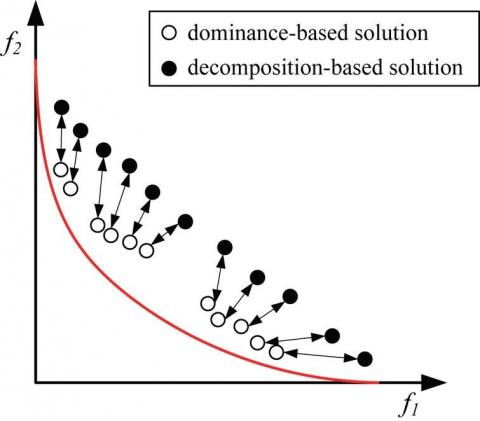Figure 1. Dominant and dominated particles of MOPSO algorithm 

Obviously, if the number of objective functions is more than 2, then the desired points will form one cube. As shown in this figure, the numbers on the Pareto front are seen in solid form and other solutions have been displayed with hollow circles. To calculate the swarm distance, i-th solution in this figure has been drawn in rectangular form that, the previous and previous solutions to the two objective functions are in its corners. The swarm distance for this point is equivalent to the average of the sides of this rectangle. The lower the swarm distance, the greater the density of the solutions is indicated. It should be noted that if the problem has more than two objective functions, i-1 and i+1 points will not be the same for all objective functions.

An appropriate selection of optimal solutions in multi-objective particle swarm algorithm is an important step, because the function is the convergence ability of the algorithm and its ability to achieve a diverse set of non-post solutions in the decision space. In the MOPSO algorithm, the limited external archive stores non-post solutions.

To find optimal solutions, the batch of particles should not be condensed in certain points to cover all the search space. For this purpose, in this method, priority is given to non-post solutions that have a greater swarm distance value. In the external archive, non-post solutions are sorted by the amount of swarm distance in descending order. Then, at each step, several high archive solutions (for example, higher 10% of the archive) are randomly selected. This descending order in the external archives elsewhere also helps us when the archive is full.

## 2.jpg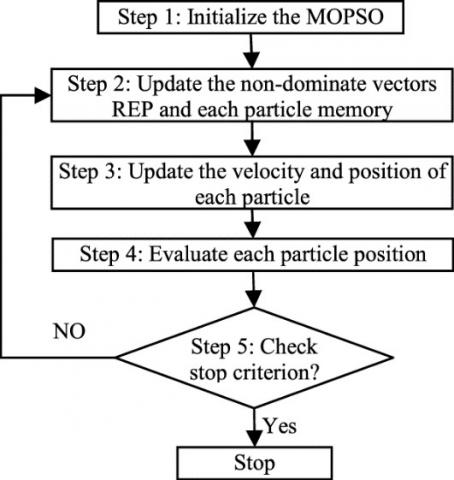Figure 2. Flowchart of MOPSO Algorithm

In such cases, low non-post archive responses (e.g., lower 10% of the archive) are randomly selected and replaces the new non-post solution that has been found in the recent iteration. The flowchart of the MOPSO algorithm is shown in Figure 2. The MOPSO algorithm performs the following process to determine the optimal location and capacity of distributed generation sources and capacitive banks simultaneously in the distribution system with the goal of reducing losses, improving the voltage profile, and reducing the cost.

Step 1: Enter the parameters of the MOPSO algorithm (number of particles, iterations, Rep, C1, C2, ...).

Step 2: Enter the network parameter (Parameters of load, lines, keys and ...).

Step 3: Particles are randomly numbered (Each of these particles represents the location and capacity of the distributed generation sources and the capacitor bank).

Step 4: The values of each particle in the objective functions are put and their values are calculated.

Step 5: Dominant Particles (Rep), accordingly, the values ​​of the best personal memories (Pbest) and the best collective memories (Gbest) are calculated.

Step 6: Afterwards, new particles are created considering the results obtained in the fifth step.

Step 7: The particle size generated are put in the objective functions and the value of each of the functions for all particles is calculated.

Step 8: If the termination conditions are not met, go to step five.

Step 9: End.

6. Simulation and Analysis of Results

In the previous section, the objective functions and constraints governing the problem of the simultaneous locating of distributed products and capacitor banks in the distribution system and how it is performed by the multi-objective particle swarm algorithm have been expressed. In this part of the paper, the results of simulations are presented in the MATLAB software environment. For this purpose, the 33-bus network has been selected IEEE standard as a sample network for conducting studies. In Figure 3, the single-line diagram of the system has been shown. This system is a radial network connected through a downstream transformer to the top-hand network.

## 3.jpg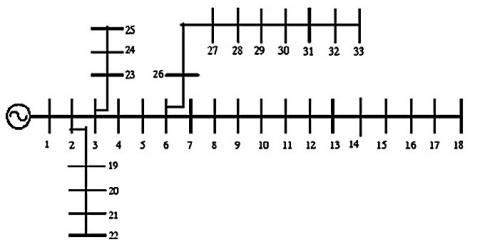Figure 3. The single-line graph of the studied network

The system has 33 buses and 32 communication lines. In the above system, bus No. 1 has been selected as the reference bus. The total active and reactive load on this network is 3.72 MW and 2.3 Mvar, respectively. The goal of optimization in this section is to find the location and capacity of capacitor banks and distributed generation sources in the studied system and with the approach to reduce losses, improve the voltage profile and reduce costs. For this purpose, two single-objective (PSO) and multi-objective (MOPSO) particle swarm algorithms were used, and the parameters of these two algorithms have been presented in Table 1.

Studies have been repeated in three scenarios. In the first scenario, loss reduction and improvement of voltage profile were selected as objective functions and the optimization was done on this basis, but in the second scenario, there was a decrease in loss functions and the cost of the agenda and improving the voltage profile was considered as a limitation of the problem. Finally, in the third scenario, the simultaneous reduction of all three objective functions has been performed simultaneously.

6.1 First scenario (loss reduction and improvement of voltage profile)

In this scenario, the objectives of the problem are to reduce the power losses in the lines and improve the bus voltage profile. Eq. (18) has been used as the objective function for locating and determining the optimal capacity of active and reactive sources by the single-objective particle swarm algorithm. This relationship is a weight function of the loss and voltage that is converted to a function.

$f=f_{1}+K_{2} f_{2}$  (18)

## 4.png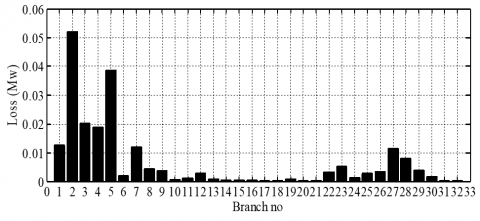Figure 4. Losses of lines in the studied network

In Eq. (18), f1 is the loss function and f2 is the voltage function that, the weighted coefficient of k2 = 0.1 has been used so that the two objective functions are equally weighed. In this relationship, the amount of losses is in kilowatt. The loss of the 33-buss distribution system before the change in the installation of distributed generation sources and capacitor banks is equal to 211.2 kilowatts. In Figure 4, the losses of each line are represented as a bar graph. The highest amount of losses is related to the pary lines that, the current of these lines is higher than the lines and the high length of these lines is another reason for their high losses.

In Figure 5, the voltage profile in different buses is shown for the 33-bus system. In the absence of active and reactive power sources, the value of the voltage function (Eq. (2)) has been calculated to be 1.8054.

In this system, the 18-bus voltage with the amplitude of 0.9037 p.u. has the lowest value. The percentage of voltage loss in this bus is about 10%, which is more than permissible limit in distribution systems (5%) that, the load of reactive and reactive power compensators must improve the voltage range of this bus. After optimization by three GA, PSO and MOPSO algorithms, the proper location and capacity for installing distributed generation sources and capacitor banks were obtained. In Figure 6 the curve of the objective function changes has been shown in various repetitions of the PSO and GA algorithms.

As shown in Figure 6, the PSO algorithm compared to genetic algorithm converges to the lower value after 27 repetitions. The final value of the objective function for the PSO algorithm is 0.167 and for the GA algorithm it is 0.183. The dominant particles curve for the MOPSO algorithm has been shown in Figure 7.

Table 1. PSO, MOPSO algorithm parameters

 $\gamma$ Mutation Rate Mutation Crossover Iteration Population GA 0.02 0.03 0.3 0.8 30 100 W Vmin Vmax C1=C2 Iteration Population PSO 0.7 0.9 0.4 2 30 100 C1=C2 Vmin Vmax Rep Iteration Population MOPSO 2 0.9 0.4 10 30 100

## 5.png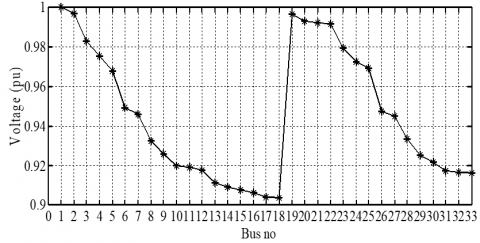Figure 5. Voltage Profile in 33-bus Network

## 6.png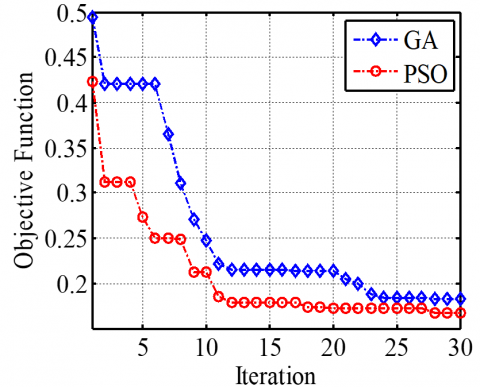Figure 6. The diagram of objective function changes

Among the dominant particles obtained by the MOPSO algorithm, the third particle was selected as the final solution. The results obtained from optimization by these three algorithms have been summarized in Table 2.

According to the results obtained in Table 2, after optimization by the GA algorithm, buses 33 and 12 have been selected for the installation of distributed generation sources each of which has a capacity of 1190 and 1300 kilowatts, respectively. Capacitor banks with capacities of 1700 and 700 KVAR are also proposed for installation in buses 6 and 8 respectively. If the PSO algorithm is used, the bus 15 with an active power capacity of 790 kilowatts, and also bus 33 with the capacity of 1210 kilowatt for the installation of DG and buses 7 and 30 for the installation of capacitor banks with capacities of 1720 and 570 KVAR, are selected to obtain the lowest value of the objective function while the MOPSO algorithm has selected buses 28 and 13 as the proper bus for the installation of distributed generation. The active power values of each distributed generations are 1370 and 1100 kilowatts, respectively, and their reactive power sources are 730 and 1340 KVAR for buses 25 and 30, respectively. In Figure 8, the losses of each of the lines have been presented for three GA, PSO and MOPSO algorithms.

The values of the objective function f1 and f2 for GA algorithms are 58.4 and 0.124, for the PSO algorithm are 46.6 and 0.120, and for the MOPSO algorithm are equal to 41.3 and 0.098. The values of the two objective functions for the MOPSO algorithm are less than two other single-objective algorithms which demonstrates the precise and accurate performance of this algorithm in minimizing simultaneously both of the objective functions. Figure 8 below shows the power losses of all lines after locating and the determination of capacity of distributed generation sources.

In Figure 8, the red line is associated with the GA algorithm, the green dot line is associated with the PSO algorithm and the black line is associated with the MOPSO algorithm. As you can see, the line losses for the MOPSO algorithm are less than two other algorithms on most lines. In the following, in Figure 9, the voltage curve of each of the 33 buses studied for the three algorithms has been shown.

## 7.pngFigure 7. Dominant values in the first scenario

Table 2. Results of optimization in the first scenario

 f2 (\$) Loss reduction % f1 (kw) Vworst Capacitor II Capacitor I DG II DG I Capacity (Mvar) Bus No Capacity (Mvar) Bus No Capacity (MW) Bus No Capacity (MW) Bus No 1.8 0 211 0.9 - - - - - - - - Base 0.124 72.3 58.4 0.982 0.7 8 1.7 6 1.3 12 1.19 33 GA 0.12 77.9 46.6 0.983 0.57 30 1.72 7 1.21 33 0.79 15 PSO 0.098 80.4 41.3 0.991 1.34 30 0.73 25 1.10 13 1.37 28 MOPSO ## 8.png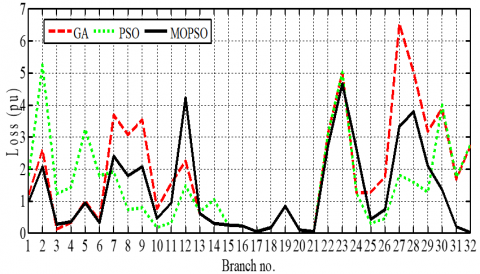Figure 8. The amount of line losses in the first scenario ## 9.png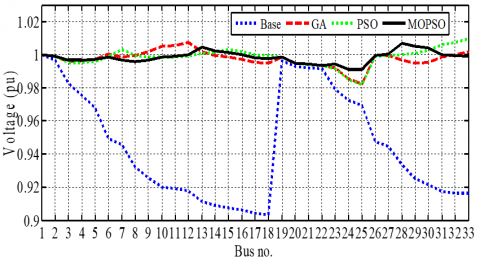Figure 9. Bus voltage range in the 33-bus network in the first scenario As shown in Figure 9, network voltage profile has been improved dramatically after installing distributed generation and capacitor banks. So, after installing the sources, the voltage range in any of the buses is less than 0.98 per unit. 6.2 Second scenario (loss and cost reduction) In this scenario, the problem of reducing the power loss of lines along with the cost reduction of the subject has been addressed. The cost per kWh of the active power lost in the transmission line is \$ 0.8. The cost of producing each kilowatt of distributed generation power was \$0.8 and the cost per KVAR of the reactive power of the capacitor bank was \$ 0.6. The costs for capacitor banks and distributed generations include the cost of initial investment, maintenance and replacement costs. Eq. (19) has been used as the objective function to determine the optimal location and capacity of active and reactive sources by single-objective particle swarm and genetic algorithms. This relationship is a weighting function of losses and costs that has become a function.

$f=f_{1}+K_{3} f_{3}$  (19)

where, f1 is the loss function and f3 is the cost function with a weight coefficient of k3 = 0.0001. In this relationship, the amount of loss us in megawatts and the cost in dollars. It should be noted that the voltage range has been considered as a constraint in the optimization problem.

$0.97 \leq\left|v_{i}\right| \leq 1.05$  (20)

The voltage of all buses should remain within the range of 0.97 to 1.05 per unit. After optimization by three GA, PSO and MOPSO algorithms, the appropriate location and capacity for installing distributed generation sources and capacitor banks were obtained. In Figure 10, the curve of the objective function changes has been shown in various repetitions of the PSO and GA algorithms.

## 10.png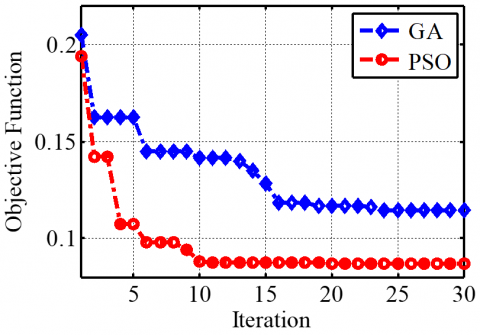Figure 10. The curve of the objective function changes in the second scenario

## 11.png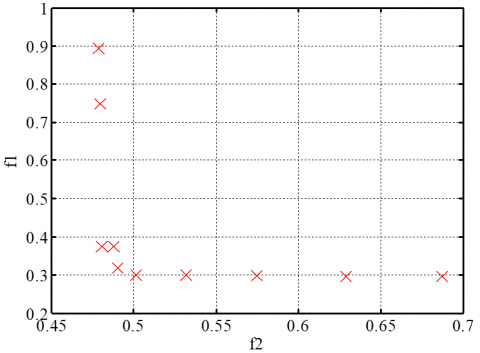Figure 11. Dominant values in the second scenario

The PSO algorithm after 19 replicates reached its final value of 0.086, and the GA algorithm converged to 0.114 after 18 replicates. The dominant particle curve for the MOPSO algorithm has been shown in Figure 11.

In the Table 3, the optimization results have been presented by these three algorithms. According to the results of Table (3), if optimized by the GA algorithm, buses 23 and 12 have been proposed for the installation of distributed generation sources, with a capacity of 859 and 975 kilowatts, respectively. Capacitor banks with capacities of 1577 and 710 kV are respectively selected for installation in buses 6 and 2.

Table 3. Results from optimization in the second scenario

 f2 (\$) Loss reduction (%) f1 (kw) Vworst Capacitor II Capacitor I DG II DG I Capacity (Mvar) Bus No Capacity (Mvar) Bus No Capacity (MW) Bus No Capacity (MW) Bus No 1690 0 211 0.9 - - - - - - - - Base 645 76.7 49 0.982 0.71 2 1.577 6 0.975 12 0.859 33 GA 523 83.9 34 0.974 0.984 7 0.596 33 1.085 30 0.834 13 PSO 487 84.3 32 0.975 0.888 30 0.637 26 0.977 12 0.845 33 MOPSO If the PSO algorithm is used, the bus 13 with an active power capacity of 834 kilowatts and bus 30 with the capacity of 1085 kilowatts for installing distributed generation and buses 33 and 7 for the installation of capacitor banks with capacities of 596 and 984 kvar are proposed to minimize the objective function. The MOPSO algorithm has proposed the buses 33 and 12 as the selected buses for the installation of distributed generation with capacities of 845 and 977 kW, as well as capacity of capacitor banks of 637 and 888 kvar for buses 26 and 30. The loss of the distribution system in the case of using GA, PSO and MOPSO algorithms is 49, 34 and 32 kilowatts, respectively. The cost of the system has been calculated at \$ 1690 in the absence of active and reactive sources, which is estimated at 645 for the GA algorithm, \$523 for the PSO algorithm and \$ 487 for the MOPSO algorithm. It should be noted that the minimum voltage range for the distributed distribution system has been obtained whose values for the GA algorithm is 0.982 and for the PSO and MOPSO algorithms were respectively 0.974 and 0.975, that any value obtained in the condition of the problem applies.

## 12.png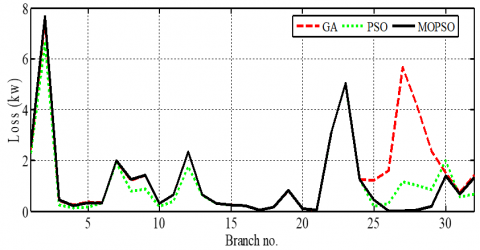Figure 12. The amount of line losses in the second scenario

## 13.png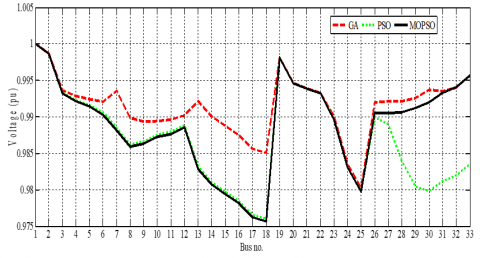Figure 13. Bus voltage range in the second scenario

In Figure 12, the power losses of all lines after locating and the determination of the capacity of distributed generation sources have been presented. As shown in Figure 12, the loss of lines for the MOPSO algorithm is less than two other algorithms in most lines and the power losses in just a few lines are slightly greater than the other two algorithms. In Figure 13, the voltage curve of each of the 33 buses studied for the three algorithms has been shown.

6.3 Third scenario (simultaneous reduction of losses and costs and improvement of voltage profile)

In this scenario, the simultaneous minimization of line power and cost losses and improvement of the voltage profile have been studied and simulated. In order to determine the optimal location and size of distributed generation sources and capacitor banks, using single-objective particle swarm and genetic algorithms, relationship (21) is used as the objective function. This relationship is a weighting function of losses, costs, and voltage profiles that have created a hybrid function.

$f=f_{1}+K_{2} f_{2}+K_{3} f_{3}$  (21)

In relationship (21), f1 is the loss function, f2 is the voltage profile function and f3 is the cost function in which the coefficients k2 = 0.1 and k3 = 0.0001 are considered. It should be noted that in this relationship the loss is in megawatts and the cost in dollar.

After determining the optimal location and size of active and reactive sources by three GA, PSO and MOPSO algorithms, the appropriate location and capacity of this installation were obtained. In Figure 14, the graph of changes in the objective function (relationship 21) has been shown in various repetitions for the PSO and GA algorithms.

## 14.png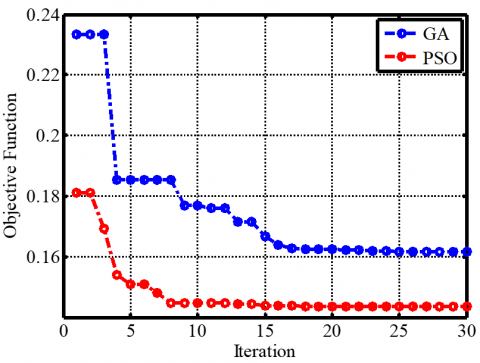Figure 14. The curve of the objective function changes in the third scenario

## 15.png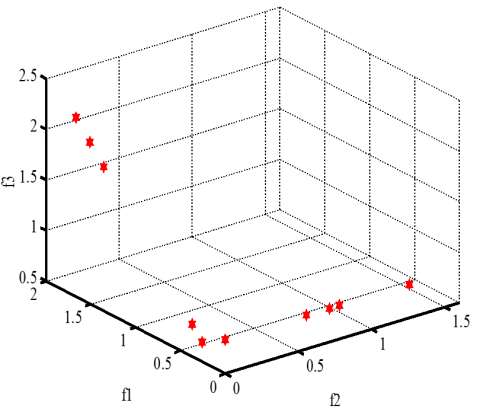Figure 15. Dominant values in the third scenario

As shown in Figure 14, the particle swarm algorithm converges to 0.1435 after 15 repetitions while the genetic algorithm has reached 0.1616 after 25 repetitions. In this scenario, the PSO algorithm has also been able to reduce the value of the objective function compared to the genetic algorithm. The dominant particle curve for the MOPSO algorithm has been shown in Figure 15.

In the Table 4, the results of optimization have been presented by these three algorithms. According to the results of Table 4, in the case of optimization by genetic algorithm, the bus 33 with a capacity of 1.32 MW and the bus 12 with a capacity of 845 kW are proposed for the installation of distributed generation sources. Capacitor banks with a capacity of 845 kVar at bus 8 and 710 kVar at bus 3 have been proposed. But if the PSO algorithm is used, the bus 15 with a power capacity of 1.125 MW and the bus 32 with a power of 885 kW for installation of distributed generations and the bus 27 with the capacity of 2740 kVar and bus 18 with a capacity of 1120 kVar for the installation of capacitive banks have been proposed to minimize the objective function. While the MOPSO algorithm has selected buses 26 and 12 as selected buses for the installation of distributed generation with capacities of 932 and 1210 kW, as well as capacitor banks with the capacitor 720 and 850 kVar for buses 27 and 30. In this case, the magnitude of the ohmic losses of the distribution system for GA, PSO and MOPSO algorithms was 76.9, 63.7, and 47.3 kw, respectively. The value of the voltage function for the GA algorithm is equal to 0.153, for the PSO algorithm is 0.167 and for the MOPSO algorithm is equal to 0.132. The total cost of the system for the case of active and reactive sources is set at \$694 for active GA and \$ 694 for the GA algorithm, \$631 for the PSO algorithm and \$ 540 for the MOPSO algorithm. According to Figure 16, it can be said that the value of the most lines of loss in the case of using the MOPSO algorithm is less than two other algorithms. In the following, in Figure 17, the voltage range of each of 33 buses of 33-bus network has been shown for three algorithms.

## 16.png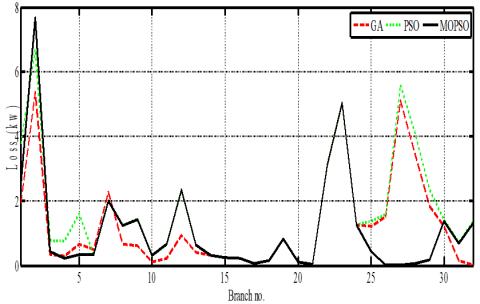Figure 16. The amount of lines loss in the third scenario

## 17.png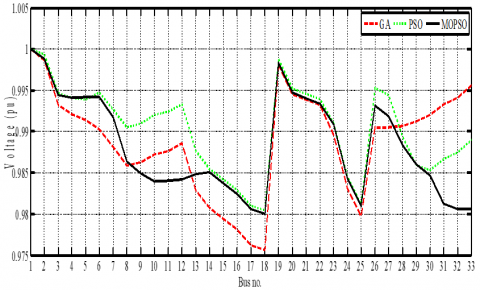Figure 17. Bus voltage range in the third scenario

The minimum value of the voltage range for the distributed distribution system is obtained whose values for the GA algorithm were 0.975 and for the PSO and MOPSO algorithms, respectively, equal to 0.981 and 0.98 respectively.

Table 4. Results from optimization in the third scenario

 f3 (\$) f2 Loss reduction (%) f1 (kw) Capacitor II Capacitor I DG II DG I Capacity (Mvar) Bus No Capacity (Mvar) Bus No Capacity (MW) Bus No Capacity (MW) Bus No 1690 1.8 0 211 - - - - - - - - Base 694 0.153 63.55 76.9 0.890 3 1.320 8 0.845 12 1.32 33 GA 631 0.167 69.81 63.7 1.120 18 0.640 27 0.885 32 1.125 15 PSO 540 0.132 77.58 47.3 0.850 30 0.720 27 1.210 12 0.932 26 MOPSO 6.4 Comparison of simulation results in different scenarios This section examines the simulation results. For this purpose, three GA, PSO and MOPSO algorithms were used to determine the location and capacity of distributed generation sources and capacitor banks with the goal of reducing losses, improving the voltage profile and reducing costs in the 33-bus network. The studies were repeated in three scenarios. In the first scenario, the loss function and voltage profile were selected as objective functions and locating and determination of active and reactive resource capacity by algorithms. In order to verify the performance of each algorithm, the losses and the voltage function in this scenario have been represented as a bar graph in Figure 18. In Figure 18, f1 shows the value of the loss function and f2 the value of the voltage function. As shown in the figure, after the installation of distributed generation and capacitor banks in the studied system, the losses are up to 75% and the voltage function has been improved up to more than 93%. The loss of the minimum value of the loss function and voltage have been obtained for the MOPSO algorithm and this algorithm has managed to keep these two functions at its minimum. In Figure 19, the results obtained in the second scenario have been compared by the algorithms. In this scenario, two loss and cost functions were selected as objective functions and the voltage function has been considered as the problem constraint. In Figure 19, f1 is the value of loss function and f2 is the value of the cost function. Considering the values of the objective functions in the second scenario, one can infer that the distribution system is better than two other algorithms in the optimal locating of capacitor banks and distributed generation sources by the MOPSO algorithm and has been able to make the lowest losses at the lowest cost. Although the loss of this algorithm is approximately equal to the PSO algorithm but it has lower cost. But in the third scenario, losses and costs were reduced and the voltage profile improved simultaneously. ## 18.png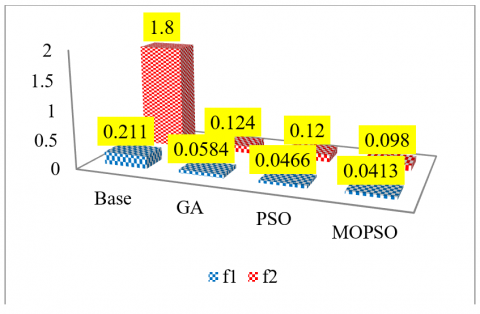Figure 18. The values of objective functions in the first scenario ## 19.png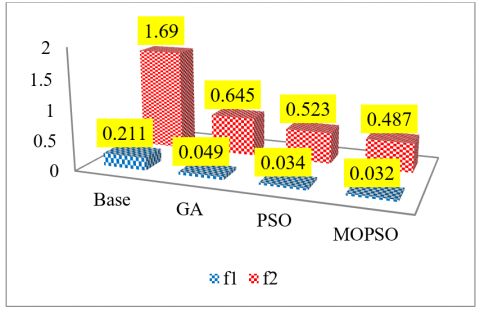Figure 19. The values of objective functions in the second scenario In Figure 20, the values of the objective function have been represented as a bar graph. In the following, in Figure 21, the percentage reduction in losses in two scenarios is shown as a bar graph. As shown in Figure 21, the percentage of loss reduction in the second scenario is higher than the first scenario. The percentage of loss reduction in the first scenario for three GA, PSO and MOPSO algorithms was calculated to be 72.3, 77.9, and 80.4, respectively while these values in the second scenario for the three algorithms are 76.7, 83.9 and 84.3, respectively. The reason for the decrease in the losses in the first scenario compared to the second scenario is the objective function defined for it. In the first scenario, in addition to reducing losses, the voltage profile was also improved which has prevented further loss. In the third scenario, the loss reduction percentage for these three algorithms is 63.55, 69.81 and 77.58, respectively. ## 20.png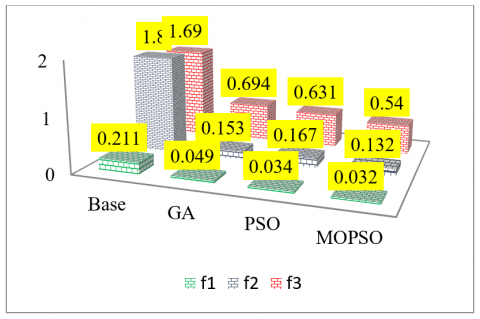Figure 20. The values of objective functions in the third scenario ## 21.jpg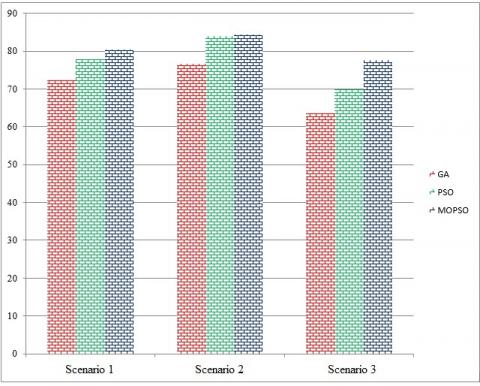Figure 21. Percentage of loss reduction 7. Conclusion In recent years, the growth trend of distribution networks in developing countries has increased dramatically. However, due to the lack of proper design of these systems, significant amounts of electrical energy are lost in them. Energy losses in power networks impose huge sums of money on electricity companies. The cost of losses includes losses, in addition to income reduction, investment costs for the creation of new facilities. The constraints on energy resources and costly energy transfer have encouraged subscribers, engineers, designers and researchers in the electricity distribution industry to increase efficiency and reduce network losses over the past decade. In other words, a further decrease in the losses leads to an increase in capital. Hence, loss reduction is an economic activity and reduces the cost of installing additional equipment in the network, the rate of energy and power loss, carbon dioxide emission rate and other economic parameters. About 75% of the network losses occur in the distribution sector, so losses in distribution systems are of great importance. Reconstruction, capacitor placement and the use of distributed generation sources are the effective ways to reduce losses and improve the voltage profile in distribution networks, which is drawn nowadays much more attention. If distributed generation sources and capacitor banks are simultaneously installed in distribution networks at the right location with optimal capacity, this will improve the technical and economic parameters of this network. In this paper, genetic algorithms (GAs), particle swarm optimization (PSO) and multi-objective particle swarm optimization (MOPSO) have been used to determine the capacity of distributed generation sources and capacitor banks in the standard 33-bus distribution network. Simulations have been conducted in two scenarios. In the first scenario, the losses and voltage profiles were considered as two objective functions used in the algorithms. After optimization by the GA algorithm, the value of the objective function of loss and voltage are respectively 58.4 and 0.124, and in the case of optimization by PSO algorithm are 46.6 and 0.120 respectively, and for the MOPSO algorithm are 41.3 and 0.098. The values of the two objective functions for the MOPSO algorithm are less than two other single-objective algorithms which demonstrates the precise and accurate performance of this algorithm in minimizing simultaneously both of the objective functions. In this scenario, after installing distributed generations and capacitor banks in the studied system, losses have been improved up to 75% and voltage function up to 93%. In the second scenario, the reduction in losses and costs have been investigated simultaneously. For this purpose, determining the optimal location and capacity of active and reactive sources were performed by three algorithms. The loss of the distribution system in the case of using GA, PSO and MOPSO algorithms is 49, 34 and 32 kilowatts, respectively. The cost of the system was \$ 1,690 if the active and reactive sources were not installed that, this is obtained after installing resources at \$645 for the GA algorithm, \$ 523 for the PSO algorithm and \$487 for the MOPSO algorithm. It should be noted that the minimum voltage range for the distribution system has been obtained whose values for the GA algorithm equal to 0.982, and for the PSO and MOPSO algorithms, respectively, equal to 0.974 and 0.975, respectively, that any resulting value is true in the condition of the problem. In the third scenario, the loss and cost reduction, and also the improvement of the voltage profile, are simultaneously addressed. Locating and determining the optimal capacity of active and reactive resources was carried out by three algorithms. The magnitude of ohmic losses in the distribution system studied for GA, PSO and MOPSO algorithms was 76.9, 63.7, and 47.3 kw, respectively. The value of the voltage function for the GA algorithm is equal to 0.153, for the PSO algorithm is 0.167 and for the MOPSO algorithm is equal to 0.132. The system costs have been calculated in the case of installation of distributed generation sources and the capacitor bank at \$ 694 for the GA algorithm, \$631 for the PSO algorithm and \$ 540 for the MOPSO algorithm.

References

 Gallego, R.A., Monticelli, A., Romero, R. (2001). Optimal capacitor placement in radial distribution networks. IEEE Transactions on Power Systems, 16(4): 630-637. 10.1109/59.962407

 Keane, A., Malley M.O. (2005). Optimal allocation of embedded generation on distribution networks. IEEE Transaction Power Systems, 20(3): 1640- 1646. 10.1109/TPWRS.2005.852115

 Gomez, G., Lopez, M.A., Jurado, F. (2012) Optimization of distributed generation systems using a new discrete PSO and OPF. Electric Power Systems Research, 84(1): 174-180. https://doi.org/10.1016/j.epsr.2011.11.016

 Moradi, M.H., Abedini, M. (2012). A combination of genetic algorithm and particle swarm optimization for optimal DG location and sizing in distribution systems. Electrical Power and Energy Systems, 34(1): 66-74. https://doi.org/10.1016/j.ijepes.2011.08.023

 Murty, V.V.S.N., Kumar, A. (2015). Optimal placement of DG in radial distribution systems based on new voltage stability index under load growth. International Journal of Electrical Power & Energy Systems, 69: 246-256. https://doi.org/10.1016/j.ijepes.2014.12.080

 Sultana, S., Roy, P.K. (2014). Multi-objective quasi-oppositional teaching learning based optimization for optimal location of distributed generator in radial distribution systems. International Journal of Electrical Power & Energy Systems, 63: 534-545. https://doi.org/10.1016/j.ijepes.2014.06.031

 Kansal, S., Kumar, V., Tyagi, B. (2013). Optimal placement of different type of DG sources in distribution networks. International Journal of Electrical Power & Energy Systems, 53: 752-760. https://doi.org/10.1016/j.ijepes.2013.05.040

 Srinivasa, R., Ravindra, K., Satish, K., Narasimham, S.V.L. (2013). Power loss minimization in distribution system using network reconfiguration in the presence of distributed generation. IEEE Transaction Power System, 28(1): 317-325. https://doi.org/10.1109/TPWRS.2012.2197227

 Jamil, M., Anees, A.S. (2016). Optimal sizing and location of SPV (solar photovoltaic) based MLDG (multiple location distributed generator) in distribution system for loss reduction, voltage profile improvement with economic benefits. Energy, 103: 231-239. https://doi.org/10.1016/j.energy.2016.02.095

 Kayal, P., Chanda, C.K. (2013). Placement of wind and solar based DGs in distribution system for power loss minimization and voltage stability improvement. Electrical Power and Energy Systems, 53: 795-809. https://doi.org/10.1016/j.ijepes.2013.05.047

 Hung, D.Q., Mithulananthan, N. (2013). Multiple distributed generators placement in primary distribution networks for loss reduction. IEEE Trans Ind Electr, 60(4): 1700-1708. https://doi.org/10.1109/TIE.2011.2112316

 Othaman, M.M., El-Khattam, W., Hegazy, Y.G., Abdelaziz, A.Y. (2016). Optimal placement and sizing of voltage controlled distributed generators in unbalanced distribution networks using supervised firefly algorithm. Electrical Power and Energy Systems, 82: 105-113. https://doi.org/10.1016/j.ijepes.2016.03.010

 Chithra Devi, S.A., Lakshminarasimman, L., Balamurugan, R. (2017). Stud Krill herd Algorithm for multiple DG placement and sizing in a radial distribution system. Engineering Science and Technology, an International Journal, 20(2): 748-759. https://doi.org/10.1016/j.jestch.2016.11.009

 Doulan, L., Abrundean, M., Bica, D. (2016). Optimal location of a distributed generator for power losses improvement. 9th International Conference Interdisciplinarity in Engineering, 22: 734-739. https://doi.org/10.1016/j.protcy.2016.01.032

 Eyad, S.O., Abdelsalam, A.A., Abdel-Wahab, M.N., El-Saadawi, M.M. (2017). Distributed generations planning using flower pollination algorithm for enhancing distribution system voltage stability. Ain Shams Engineering Journal, 8(4): 593-603. https://doi.org/10.1016/j.asej.2015.12.001

 Civanlar, S., Grainger, J.J. (1985). Volt/var control on distribution systems with lateral branches using shunt capacitors and voltage regulators— Parts I, II and III. IEEE Transactions on Power Apparatus and Systems, PAS-104(11): 3278-3297. https://doi.org/10.1109/TPAS.1985.318844

 Baran, M.E., Wu, F.F. (1989). Optimal capacitor placement on radial distribution systems. IEEE Transactions on Power Delivery, 4(1): 725-733. https://doi.org/10.1109/61.19265

 Huang, Y.C., Yang, H.T., Huang, C.L. (1996). Solving the capacitor placement problem in a radial distribution system using tabu search approach. IEEE Transactions on Power Systems, 11(4): 1868-1873. 10.1109/59.544656

 Sundhararajan, S., Pahwa, A. (1994). Optimal selection of capacitor for radial distribution systems using a genetic algorithm. IEEE Transactions on Power Systems, 9(3): 1499-1507. 10.1109/59.336111

 Lee, C.S., Hultmann Ayala, H.V., Santos Coelho, L. (2015). Capacitor placement of distribution systems using particle swarm optimization approaches. International Journal of Electrical Power & Energy Systems, 64: 839-851. https://doi.org/10.1016/j.ijepes.2014.07.069

 Devabalaji, K.R., Ravi, K., Kothari, D.P. (2015). Optimal location and sizing of capacitor placement in radial distribution system using Bacterial Foraging Optimization Algorithm. International Journal of Electrical Power & Energy Systems, 71: 383-390. https://doi.org/10.1016/j.ijepes.2015.03.008

 Taher, S.A., Bagherpour, R. (2013). A new approach for optimal capacitor placement and sizing in unbalanced distorted distribution systems using hybrid honey bee colony algorithm. Int J Electr Power Energy Syst, 49: 430-448. https://doi.org/10.1016/j.ijepes.2013.02.003

 Satish, K.I., Vinod, K.T., Meera, S.H. (2015). Optimal allocation of capacitor banks in radial distribution systems for minimization of real power loss and maximization of network savings using bio-inspired optimization algorithms. International Journal of Electrical Power & Energy Systems, 69: 441-455. https://doi.org/10.1016/j.ijepes.2015.01.040

 Mohamed Shuaib., Y., Surya Kalavathi, M., Christober, Asir Rajan, C. (2015). Optimal capacitor placement in radial distribution system using Gravitational Search Algorithm. International Journal of Electrical Power & Energy Systems, 64: 384-397. https://doi.org/10.1016/j.ijepes.2014.07.041

 Sneha, S., Provas, K.R. (2014). Optimal capacitor placement in radial distribution systems using teaching learning based optimization. International Journal of Electrical Power & Energy Systems, 54: 387-398. https://doi.org/10.1016/j.ijepes.2013.07.011

 Sajjadia, S.M., Haghifam, M.R., Salehi, J. (2013). Simultaneous placement of distributed generation and capacitors in distribution networks considering voltage stability index. International Journal of Electrical Power & Energy Systems, 46: 366-375. https://doi.org/10.1016/j.ijepes.2012.10.027

 Gopiya Naik, S., Khatod, D.K., Sharma, M.P. (2013). Optimal allocation of combined DG and capacitor for real power loss minimization in distribution networks. International Journal of Electrical Power & Energy Systems, 53: 967-973. https://doi.org/10.1016/j.ijepes.2013.06.008

 Gallano, R.J.C., Nerves, A.C. (2014). Multi-objective optimization of distribution network reconfiguration with capacitor and distributed generator placement. TENCON 2014- IEEE Conference Region, 10: 1-6. https://doi.org/10.1109/TENCON.2014.7022365

 Neeraj, K., Nikhi,l G., Niazi, K.R., Swarnkar, A. (2015). Improved meta-heuristic techniques for simultaneous capacitor and DG allocation in radial distribution networks. International Journal of Electrical Power & Energy Systems, 73: 653-664. https://doi.org/10.1016/j.ijepes.2015.05.049

 Niknam, T., Kavousifard, A., Aghaei, J. (2012). Scenario-based multi objective distribution feeder reconfiguration considering wind power using adaptive modified particle swarm optimization. IET Renew Power Gen, 6(4): 236-247. https://doi.org/10.1049/iet-rpg.2011.0256

 Coello Coello, C.A., Lechuga M.S. (2002). MOPSO: A proposal for multiple objective particle swarm optimization. IEEE Proceedings of the Congress on Evolutionary Computation CEC '02, pp. 1051-1056. https://doi.org/10.1109/CEC.2002.1004388

 Hu, Z., Yang, J., Cui, H., Sun, H., Wei, L. (2017). Multi-objective particle swarm optimization algorithm based on leader combination of decomposition and dominance. Journal of Intelligent & Fuzzy Systems, 33(3): 1577-1588.